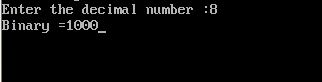UncleCoder.com

UncleCoder.com

Free programming examples and instructions

# C program to convert decimal to binary

## C program for how to convert decimal to binary

by Krishna

Posted on 27 Jun 2018 Category: C Views: 1555

C program to convert decimal to binary

``````#include <stdio.h>

void decToBinary(int);

int main()
{
int number;

printf("Enter the decimal number: ");
scanf("%d", &number);

decToBinary(number);
return 0;
}

void decToBinary(int num)
{
if (num == 0)
{
return ;
}

decToBinary(num / 2);
printf("Binary=%d", num % 2);
}``````

OUTPUT